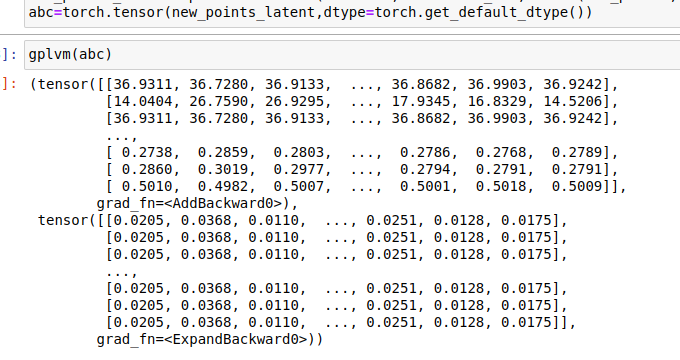# Prediction of data points using GPLVM

Hello there,
I am just starting to use pyro. I am supposed to use GPLVM and predict similar points as of my datasets. I have data of size 78 dimension, following the example given in examples/gplvm.html in pyro I reduced the dimension of data into latent space of dimension 2.
Now I need to predict similar points into latent space and back to 78 dimension. I am stuck here,(prediction of points and back to input dimension) can you please suggest how this is possible?

I feel that you need to do inference using GPLVM again on the new data points (using set_data method). But this time you can skip optimizing the GP parameters.

I am absolute beginner and hope you can further help me as I was not clear on what you mean.
what I did till now is made a kernel using kernels.RBF, initiated inducing points and used this:
`gplvm=gp.models.SparseGPRegression()`, guided using this `gplvm.X=pyro.nn.PyroSample(dist.Normal(X_init,0.1).to_event()) gplvm.autoguide("X",dist.Normal)` and fetched mean and deviation using `mean = gplvm.X_loc.detach().numpy() ` and `std_dev=gplvm.X_scale.detach().numpy() ` respectively. Further, used this to predict new points in latent space `new_points_latent=np.random.normal(loc=mean, scale=std_dev, size=(new_points, mean.shape))`.
Now I am confused, if I am on correct way or not, if yes how can I proceed forward and if not how can I correct myself. Sorry if I am too specific.

Sorry, I misunderstood your question. I thought you wanted to get latent values corresponding to “new” similar points in your dataset.

I think it’s better to use `VariationalSparseGP` instead and after fitting, you can do

``````gplvm(new_points_latent)
``````

I feel that you can also do

``````gplvm.model()
``````

so that you don’t have to fetch `mean`, `std_dev` and create `new_points_latent`. Let me know if it does not work.

1 Like

thankyou! will try it.

I tried it, I didn’t do `gplvm.model()` but the same mean and std. deviation method, I used `gplvm(new_points_latent)`
ofc, converted new_points_latent into tensor using torch.tensor, but got tuple of length 2, as it’s output and am not sure what did it return.
Couldn’t find anything such in documentation too!Hi @acharyafutures , you can find the documentation here Gaussian Processes — Pyro documentation

1 Like

Thankyou for your quick response, I had a look at it, but am not sure what is the tuple returned by it.
Sorry, if I am too naive.

GP(X) returns a Normal/Multinomial distribution for `y`. The returned value is mean and variance/covariance of that distribution. You can consider the mean as the prediction `y_pred`. If you want to incorporate the uncertainty, you can use the variance and draw samples from that distribution.

1 Like

Thankyou @fehiepsi , I guess I am clear now!

Hello @fehiepsi, thankyou for your help earlier, I did so and was going pretty well but I am confused now as I had `new_points_latent=np.random.normal(loc=mean, scale=std_dev, size=(new_points, 10))`
used this line to draw samples but the problem is I need to have `new_points= len(mean)` all the time .How can I predict more points?